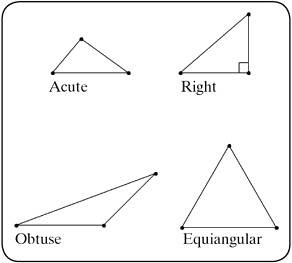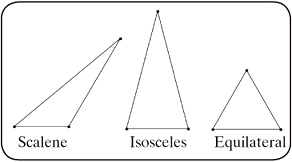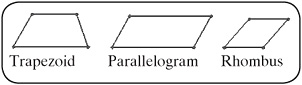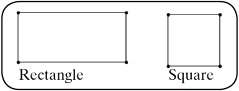Definition: polygon from Philip's Encyclopedia

Plane geometric figure having three or more sides intersecting at three or more points (vertices). They are named according to the number of sides or vertices: triangle (three-sided), quadrilateral (four-sided), hexagon (six-sided). A regular polygon is equilateral (has sides equal in length) and equiangular (has equal angles).

Summary Article: Lesson 10-1: Polygons
From Homework Helpers: Basic Math and Pre-Algebra

A polygon is a closed plane figure with at least three sides. The sides meet only at their endpoints. The point where two sides meet is called a vertex. The name of a polygon is determined by the number of sides that it has. A triangle is a polygon with three sides. A quadrilateral is a polygon with four sides. There are many different kinds of quadrilaterals. Squares, rectangles, parallelograms, and trapezoids are just a few types of quadrilaterals.

The smallest number of sides that a polygon can have is three. A three-sided polygon is called a triangle. Triangles can be classified by their angle measures. A triangle whose interior angles are all less than 90° is called an acute triangle. A triangle that has one angle that is equal to 90° is called a right triangle. A triangle that has one angle that is greater than 90° is called an obtuse triangle. A triangle whose angles all have the same measure is called an equiangular triangle. One important feature about triangles is that the sum of the interior angles is 180°. Figure 10.1 shows examples of acute, right, obtuse, and equiangular triangles.Figure 10.1.

Triangles can also be classified by the lengths of their sides. If all three sides of the triangle have different lengths, the triangle is scalene. If two sides of a triangle are the same length, the triangle is called an isosceles triangle. If all three sides of a triangle have the same length, the triangle is called an equilateral triangle. Figure 10.2 illustrates examples of scalene, isosceles, and equilateral triangles.Figure 10.2.

Moving up in the realm of polygons are the four-sided polygons, or quadrilaterals. Quadrilaterals can be classified by the relationships between their sides. Opposite sides of a quadrilateral are parallel if, upon extending the two sides into lines, the two lines never intersect. If one pair of opposite sides of a quadrilateral are parallel, the quadrilateral is called a trapezoid. If both pairs of opposite sides of a quadrilateral are parallel, the quadrilateral is called a parallelogram. Every parallelogram has the property that the sides opposite each other have the same length. If, in addition to opposite sides being the same length, adjacent sides of a parallelogram have the same length, the parallelogram is called a rhombus. Figure 10.3 shows examples of these quadrilaterals.Figure 10.3.

Quadrilaterals can also be classified by the size of their interior angles. If all four angles of a quadrilateral are right angles, then the quadrilateral is called a rectangle. If all four angles of a quadrilateral are right angles and all four sides have the same length, the quadrilateral is a square. Figure 10.4 illustrates examples of these types of quadrilaterals.Figure 10.4.

A rectangle, a rhombus, and a square are special types of parallelograms. Because they are parallelograms, they have the property that their opposite sides have the same length. When we describe a rectangle, we only need to specify the length of two adjacent sides. We call these sides the length and width of the rectangle. It doesn't matter which side we call the length and which side we call the width. We can always rotate the rectangle to give it a different orientation. Fortunately, rotating a polygon (or any shape, for that matter) doesn't change the length of the sides of the polygon. It only changes its orientation and how we see it. Of course, using the word length to represent two ideas can be confusing. We use “length” to refer to one side of a rectangle, and we use “length” when discussing measurements in general. You should understand which usage is appropriate from the context of the problem. Don't let it bother you when I talk about the length of the length of a rectangle.

With a square, we only need to specify the length of one of its sides, as all four sides have the same length. The sides of a square are referred to as just that: sides of the square.

It is easy to introduce algebra into problems that involve geometry, as we will see in the following sections. We will start with line segments and then turn our attention to triangles and other polygons.Copyright 2011 © by Denise Szecsei

### Related Articles

##### Full text Article polygon
The Penguin Dictionary of Mathematics

A figure formed by three or more points (vertices) joined by line segments (sides). The term is usually used to denote a closed plane figure in...

See more from Credo